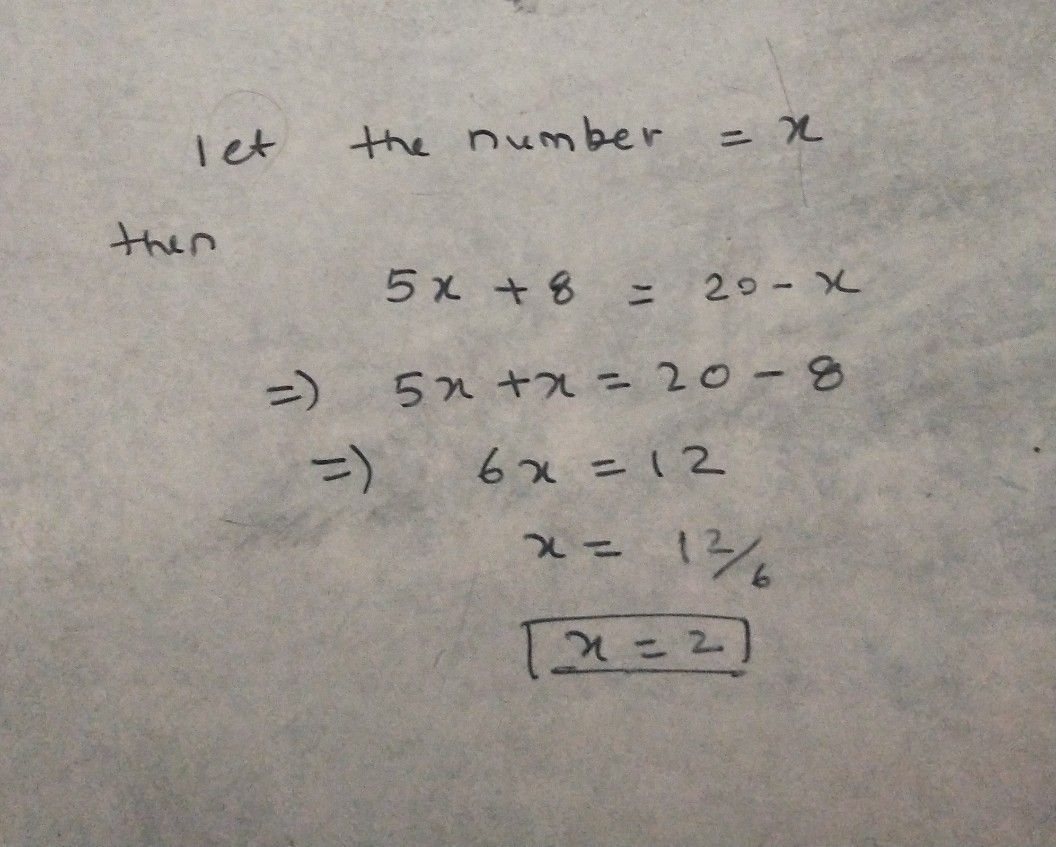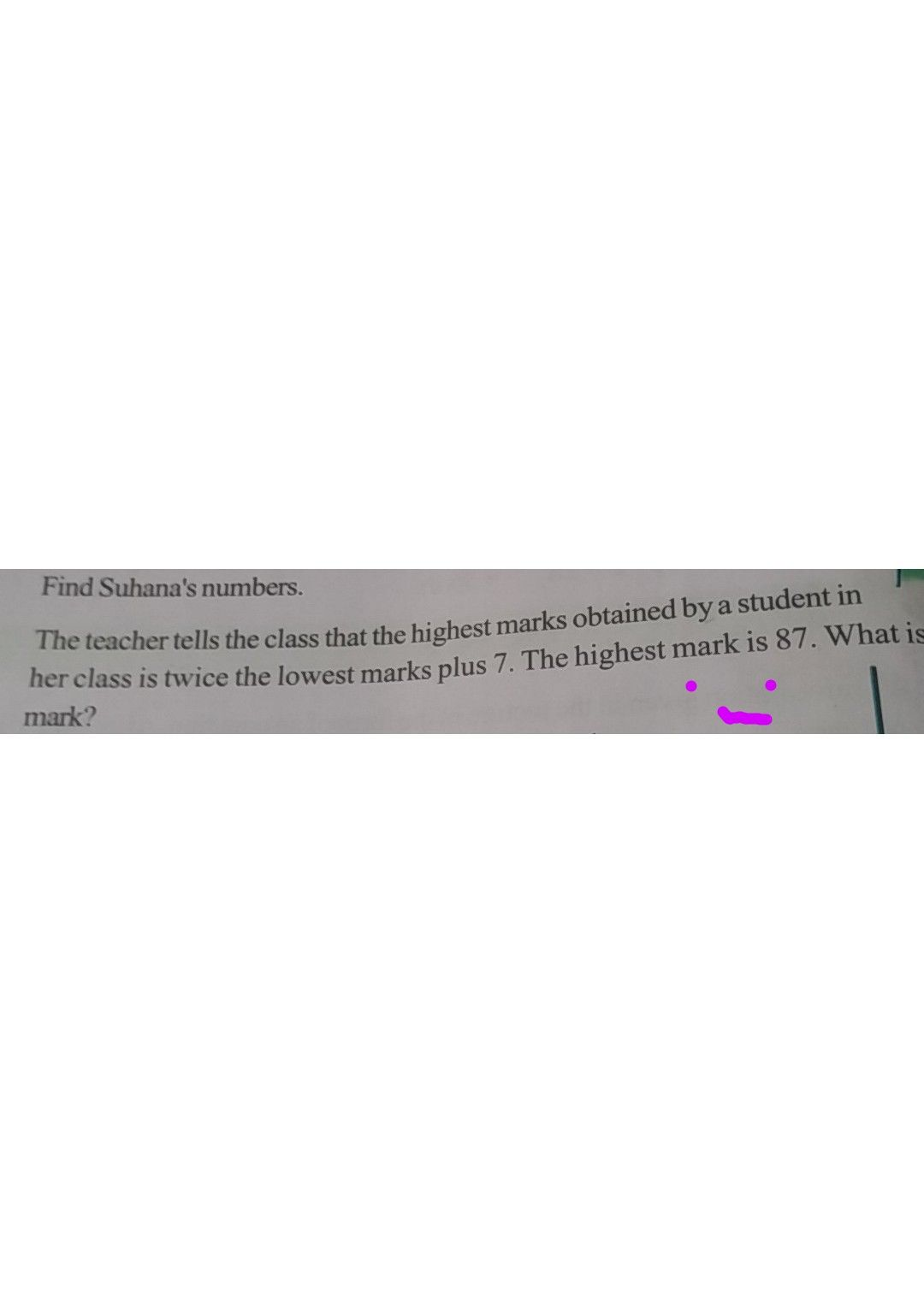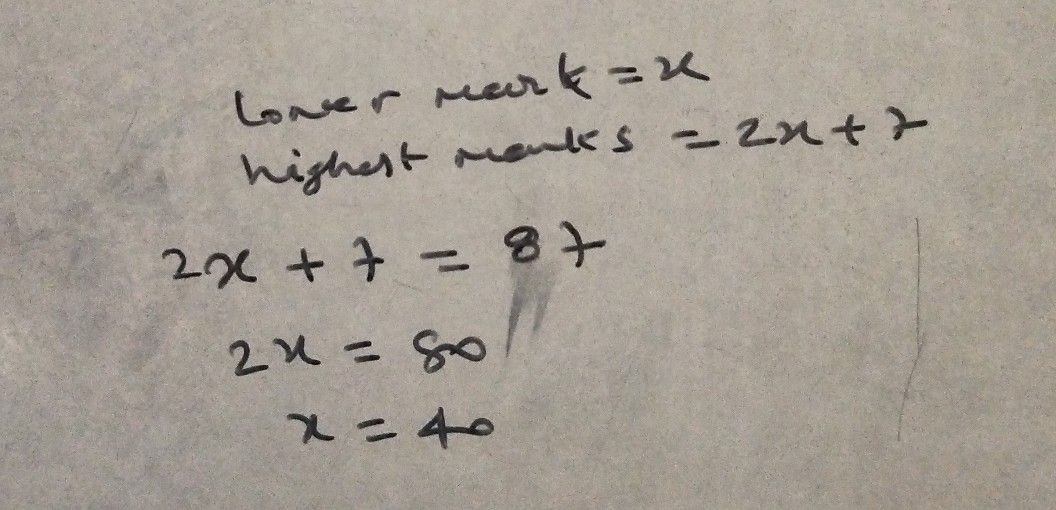Symbol
Problemparts are in the raio LLINE N Suhana said, "multiplying my number by $5a3$ adkhing Sto in gives the same answer as subtracting my number from $2y$ Find Suhana's numbers.
7th-9th grade
Geometry
Search count: 111
SolutionQanda teacher - nitinpearlStudentQanda teacher - nitinpearlyou are not able to ask again question
this is hint only
if satisfy then kindly request please don't ask question other and give feedback if satisfy
this is not good give feedback